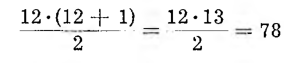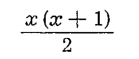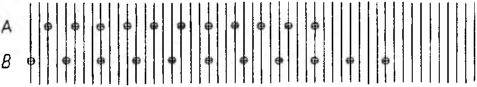# Several clock puzzlesA. How many times in a 12 hours, the minute hand will stand above the hour hand?

Twelve times? No, only eleven! For the first time, the minute hand will catch up with the hour hand at the hour 1 minutes 5 i 5/11. For each further hour, the minute hand will have to run the entire dial and 5 i 5/11 minutes. The second meeting will take place at 2 minutes 10 i 10/11, the third is at 3 16i minutes 4/11 and so on, finally the eleventh - at an hour 11 minutes 59 11/11, that is exactly at the hour 12.

B. If the wall clock strikes 6 o'clock within six seconds, how much time will it use to strike the twelfth hour?

Everyone is ready to answer at first, that he would use twelve seconds for it - forgetting, that there are five rests between the first and sixth strike, while there are six such spaces between the sixth and twelfth strokes. So the clock shall chime at twelve o'clock 13 i 1/5- seconds. We assume, that the chimes of the clock do not last, but they are instantaneous, are the moment.

C. I have - says one of the mathematicians - an old wall clock, which only strikes full hours. I have to wind it up, unfortunately, daily; I am doing it. after twelve noon. During the day, both weights of the clock are lowered equally, each fr 312 chain eyelets.
One day, having pulled up the weights of the clock, I left the house and on my return I noticed, that the clock chimed so many times, provided that one weight was higher than the other weight. It made me wonder, on the basis of that circumstance alone, could anyone find, what time did I come back. Is it a solvable task?

Yes. The weight that causes the clock to go is lowered for an hour, of course, by 312:24= 13 mesh. And how does the second sinker behave?, which falls when the clock chimes?

For twelve hours the clock strikes the hours:
1 + 2 + 3 + 4 + 5 + 6 + 7 + 8 + 9 + 10 + 11 + 12.

To quickly calculate the sum of twelve consecutive natural numbers starting with unity, it should be the last number, so the number 12, multiply by the next natural number, that is, by 13, and divide the result of the multiplication by 2.

In our case, we will getSo the clock will strike within half a day 78 times, and all day long 156 times. The weight that causes the clock to strike is lowered for each stroke of o 312 : 156 = 2 chain eyelets.
So since the clock struck x times when the owner returned, it was from twelve o'clock the clock struck beatsand during this period the weight that started the clock's chiming decreased by x(x+1) chain eyelets, while the weight moving the hands of the clock decreased by x • 13 mesh.
According to the conditions of the problem, the difference between the numbers given above was just x. Hence the equation
13 x — x (x + 1) = x

The number x is not zero. Dividing all the terms of our equation by x, we get a simpler equation:

13-(x+l) = 1,

from which we find x - 11.

The return home was at an hour 11 in the evening.

D. Two clocks A and B struck simultaneously 19 times. How to determine the time, which they pointed, as you know, that the beginning of the hour on clock A was delayed by clock B o 2 seconds and that clock A hits every 3 seconds, a clock B co 4 seconds?

Suppose, that the nth beat of clock A will coincide with the nth beat of clock B for the first time. Until then clock A has been chiming through
(n — 1) • 3 seconds, and clock B chimed through (n — 1) • 4 seconds.

We get the equation
(n — 1) • 4 — (n — 1) • 3 = 2 , from which we find n = 3.

So the third hit of A coincides with the third hit of B, and before that it was heard 2 clock strikes B i 2 a slightly lagging clock A. So if the clocks chose three o'clock, it would be audible together 5 strokes.

Over the next 12 seconds the A clock will strike 4 times, and clock B only 3 times, while over these 12 we'll hear seconds 3 separate clock strikes A i 2 separate clock beats B and 1 cumulative stroke; the seventh strike of clock A will coincide with the sixth strike of clock B. Together over the course 12 we'll hear seconds 3 + 2 +1 — 6 strokes, what about the previous ones 5 blows gives 11 strokes.

But we have to 'get there” do 19 strokes. Further 12 seconds give again 3 separate clock strikes A i 2 separate clock beats B and 1 cumulative stroke; then clock A will strike the eleventh time, and clock B for the ninth time. Together with the previous ones 11 we will hear blows 17 strokes, and then what else 4 we'll hear seconds 2 delayed clock beats B: tenth and eleventh. So it was eleven o'clock.

Here is the timing of the beats of the clocks: